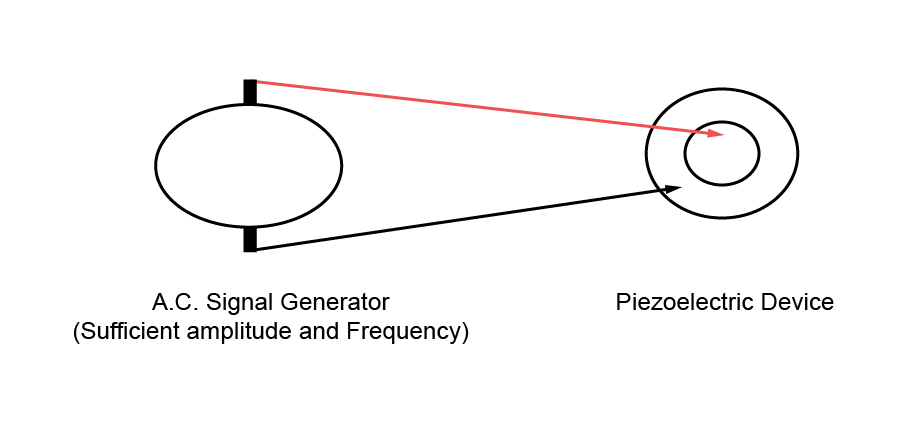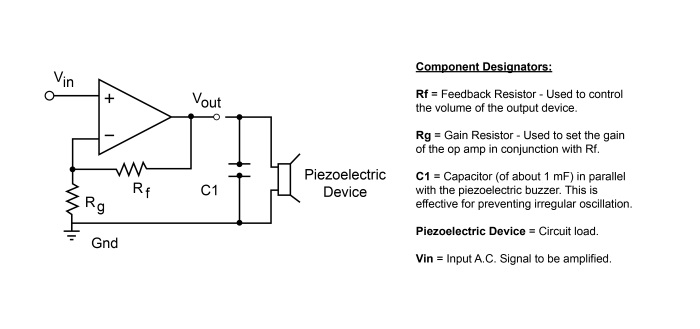# Piezo Digital Drive

## Example of a Drive Circuit for a Digital Driven Piezoelectric Device

A Digital Drive Type Circuit is defined when a solid state device such as an operational amplifier (digital component) is used to create the drive signal that drives the piezoelectric device.

In theory, a piezoelectric sounder or a piezoelectric diaphragm will emit "sound" when an AC voltage (signal) is applied to both terminals of the product. If the frequency of the AC voltage (signal) is within the hearing range of 20 Hz - 20 KHz and the amplitude of the AC voltage (signal) is large enough, the human ear will hear the buzzing sound created by the input AC voltage (signal). Below is a simple diagram of the theoretical circuit defined above:Examples of typical drive circuits are broadly divided into two types of driver circuits:

Type 1 is an analog driver circuit. An example is where analog components, such as a transistor and resistive components make up the circuit is used to provide the amplified signal to drive the device.

Type 2 is a digital driver circuit. An example is where a solid state device such as an operational amplifier (digital component) is used to create the drive signal that drives the device.

Type 2: Driven directly from an integrated circuit (Operational Amplifier).

An example of a solid state drive circuit for a piezoelectric sounder or a piezoelectric diaphragm is driven directly from an I.C. circuit, would be an operational amplifier.

A piezoelectric sound component has high impedance and is a voltage-driven device, so it can be driven directly from an IC.Please refer to the following supplementary notes regarding the components shown in the circuit diagram above.

1) For example, in a non-inverting amplifier (see the figure above) by adding negative feedback, via the voltage divider Rf and Rg, reduces the gain.

2) Equilibrium will be established when Vout is just sufficient to reach around and "pull" the inverting input to the same voltage as Vin. The voltage gain of the entire circuit is determined by 1 + Rf/Rg.

3) As a simple example, if Vin = 1 V and Rf = Rg, Vout will be 2 V, the amount required to keep V− at 1 V. Because of the feedback provided by Rf and Rg this is a closed loop circuit. Its overall gain Vout / Vin is called the closed-loop gain ACL. Because the feedback is negative, in this case ACL is less than the AOL of the op-amp.

4) In order to protect the IC from back electromotive force generated by a piezoelectric sound component, consider connecting a Zener diode in parallel with the piezoelectric sound component if necessary.

5) Design the circuit in such a way that a DC voltage is not applied to the piezoelectric sound component for a long period.